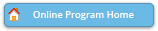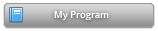#### Abstract Details

 Activity Number: 621 - Beyond Linear Regression: Nonlinear Association, Quantile Regression and Generalized Linear Models Type: Contributed Date/Time: Thursday, August 1, 2019 : 8:30 AM to 10:20 AM Sponsor: Section on Statistics in Epidemiology Abstract #302868 Title: Assessing a Multi-Prediction Model with Applications in Reproductive Endocrinology Author(s): Katharine Correia* Companies: Amherst College Keywords: zero-truncated Poisson regression; beta regression; prediction; ratio of random variables; in vitro fertilization; reproductive endocrinology Abstract: The motivating application behind this work is a collaboration with reproductive endocrinologists in which the objective is to develop a prediction tool for the number of oocytes that should be fertilized in an in vitro fertilization cycle such that enough embryos are created to yield one live birth, while minimizing the number of surplus embryos. Such a prediction is non-trivial, as it involves a non-linear function of estimates from two separate prediction models. Namely, we first estimate the number of embryos that will need to be transferred to yield one live birth (ET) using a zero-truncated Poisson regression model; then, we estimate the proportion of fertilized oocytes that will result in transferrable embryos (PF) using a beta regression model. For use in clinical practice, the final estimate is the ceiling of the ratio of these separate predictions: ceiling[ET/PF]. In this paper, we conduct a simulation study to compare the operating characteristics of this estimator using different bivariate Taylor expansions of the ratio.

Authors who are presenting talks have a * after their name.